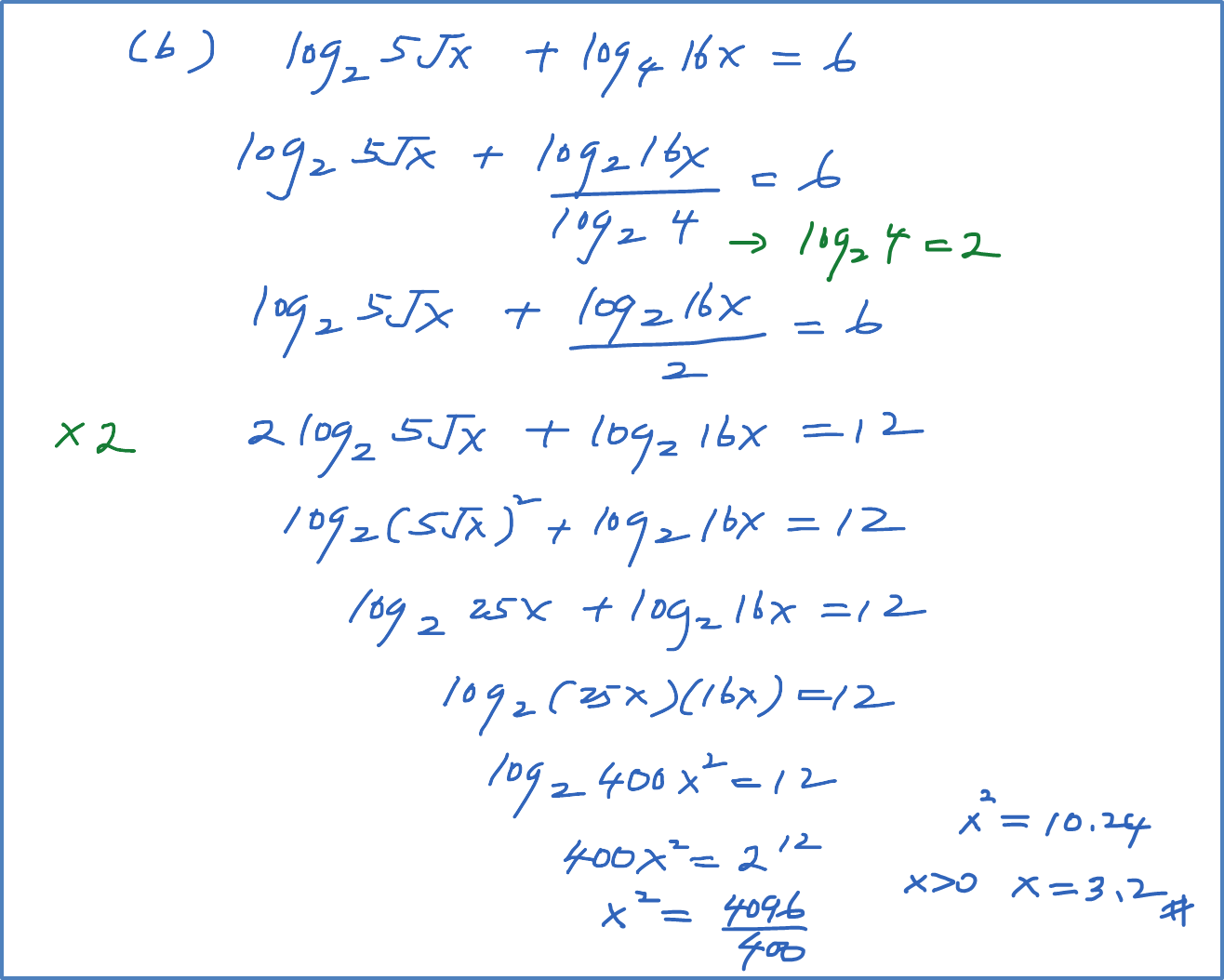\

# 4.4.4 Logarithms Equation – Example 4 & 5

Example 4
Solve the following equation :
(a) ${\mathrm{log}}_{9}\left(x-2\right)={\mathrm{log}}_{3}2$
(b) ${\mathrm{log}}_{4}x=\frac{3}{2}{\mathrm{log}}_{2}3$Example 5
Solve the following :
(a) ${\mathrm{log}}_{4}x=25{\mathrm{log}}_{x}4$
(b) ${\mathrm{log}}_{2}5\sqrt{x}+{\mathrm{log}}_{4}16x=6$